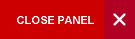## Correlation of Left Ventricular Diastolic Function and Left Ventricular Geometry in Patients with Obstructive Sleep Apnoea Syndrome

Journal Authors:
DOI:
10.7727/wimj.2014.054
Pages:
92–8

ABSTRACT

Background: The aim of this study is to evaluate the correlation of the left ventricular diastolic function and the left ventricular geometry in patients with obstructive sleep apnoea syndrome (OSAS) by echocardiography.

Methods: The 181 patients diagnosed with OSAS were divided into the normal geometry group (NG), the concentric remodelling group (CR), the eccentric hypertrophy group (EH) and the concentric hypertrophy group (CH). Pearson correlation analysis and multiple linear regression analysis were performed toward the correlation of the left ventricular diastolic function and the left ventricular geometry.

Results: The E peak in the EH and CH group was significantly reduced, with significant difference; the E/A, Em, Am and Em/Am was reduced in the order of the CR, EH and CH groups, while was E/Em was increased, and the difference was significant. Pearson correlation analysis revealed that the Em/Am showed significant negative correlations with the left ventricular mass index (LVMI) [r = -0.419] and relative wall thickness (RWT) [r = -0.289], while the E/Em was significantly positively correlated with the LVMI (r = 0.638) and RWT [r = 0.328] (p < 0.001). Multiple linear regression analysis revealed that LVMI and RWT had influence on the Em/Am and E/Em (r2 = 0.402, r2 = 0.107, p < 0.001). The left ventricular diastolic dysfunction was the worst in the CH group.

Conclusions: There was correlation between the left ventricular diastolic dysfunction and the changes in cardiac geometry.

Accepted:
01 Apr, 2014
PDF Attachment:wimjmar2015_92_98.pdf
e-Published: 03 Feb, 2015
Top of Page